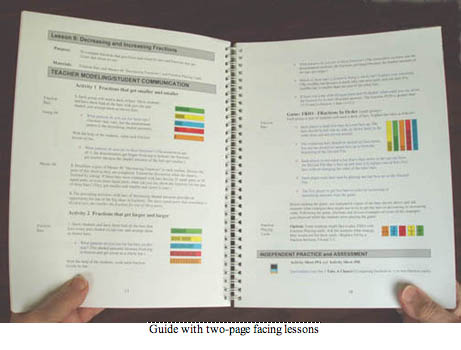Home

Grades 3-4 Fraction Bars Teacher's Guide

 Sample Lessons Grades 3-4The Grades 3-4 Fraction Bars Teacher's Guide contains lessons for each of the following topics: Fraction Terminology; Equality; Common Denominators; Inequality; Fractions for Collections; Word Problems; Number Lines; Measurement; Fractions-Decimals-Percents; Addition; Subtraction; Multiplying by Fractions; Probability and Predictions. Each lesson consists of two facing pages headed by the purpose and materials required. The main component of each lesson is TEACHER MODELING/STUDENT COMMUNICATION (instruction and student activities) and is followed by INDEPENDENT PRACTICE (activity sheets and interactive computer games). Under separate cover is the Grades 3-4 Fraction Bars Teacher Resource Package that includes Activity Sheets for each lesson and two Performance Tests. Samples of the first pages of two-page lessons from the Grades 3-4 Teacher's Guide are linked below for each of the following six topics.